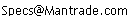Daily Speculations The Web Site of Victor Niederhoffer and Laurel KennerThe Chairman Victor Niederhoffer About Victor Niederhoffer
Write to us at:(address is not clickable)

6/2/2005
Variations on Tree Form, by Victor Niederhoffer

1. Trees often can be visually separated by how wide the branches reach versus how how high they go. A typical classification is between the cones such as the firs, pines, and spruce, called a decurrent form, and the deciduous species such as the oaks and maples where the form is circular, and the lateral branches often grow almost as far as the height. A big factor that must also be considered is how narrow the spreading form is because the elm is so compact they are planted to line the streets in cities like Reverse Horn of Plentington. Stock price movement should be characterized by horizontal and vertical components, and the result should be used to predict subsequent movements in direction and volatility. A good way to characterize horizontal moves in a stock versus the vertical is to take the total absolute variation and compare that to the total algebraic variations. For example, if the moves in a stock during a week were +1, -1, +3, -2, +4,the total algebraic move was +5 and the total absolute move was 11.

Thus, 5 /11 or 45% of the total variation was positive. A little calculation shows that the statistic 4/5 x the % of total variation about the mean that is positive (x the square root of n) is approximately normally distributed with a mean of 0 and stand deviation of 1, i.e.,is a unit normal statistic. Thus, the chances are 5% that that this statistic will be above 1.65 and 2.5% that it will be above 2 due to random factors alone. Here are some results for individual Dow stocks.

Might this not be also a very useful way to comprehend and compare volatility, the way our brains are wired to understand numbers and comparisons, instead of resorting to obtaining square root of a sum of squares:

Say for example :

+1, -1, +3, -2, +4 provides an algebraic move of +5 (net movement) while the absolute move of 11 is the total movement and the ratio 5/11 is net movement / total movement in price and thus a relative measure of volatility is 1 - 5/11 = 6/11 = .5454

+1, +1, +1, +1, +1 gives +5 and 5 thus 5/5 = 1, a perfect trend and zero volatility is given as 1-1 = 0

Simple to comprehend: more volatile moves produce more fluctuation and less volatile moves produce less fluctuation away from the regression of the trend.

So Net Change in Price divided by Sum of Absolute Deviations = trending component